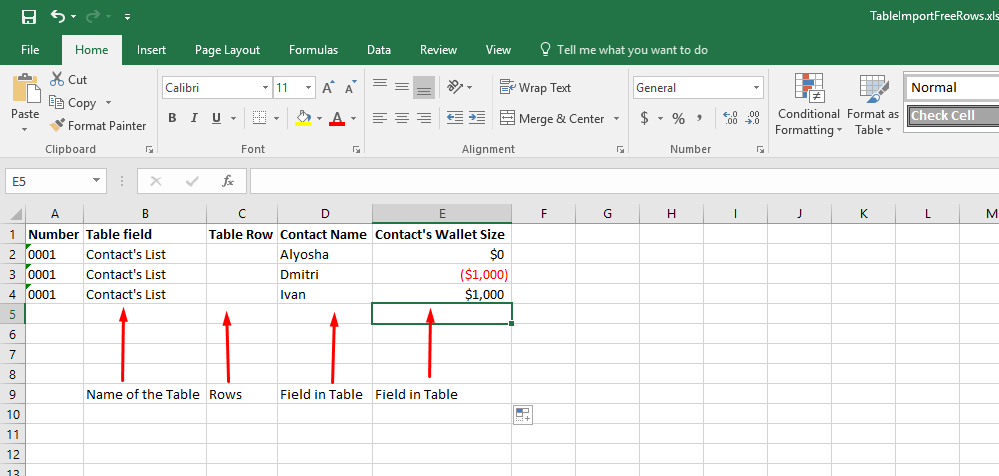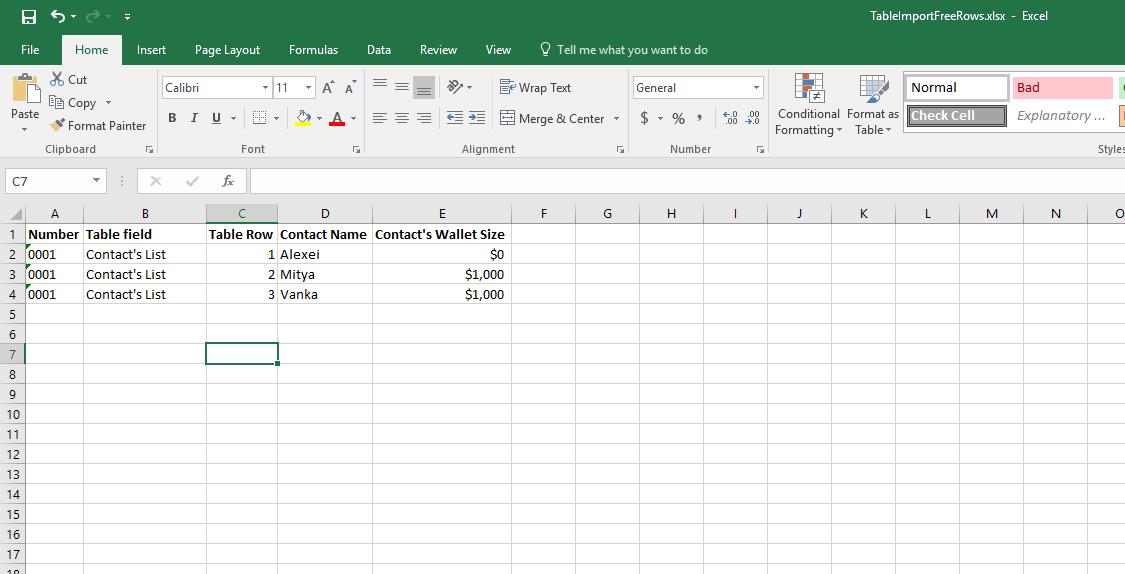There are two types of Table fields in RPM.

Free Row Tables and Defined User Row Tables

Free Row Tables are tables that can have as many rows as the User entering data into a Form would want. While a Defined User Row Table has a set amount of rows as defined by the user who created the table. The process of importing into these tables are unique to each.

If you're unsure which Table your Table is check a Form where the table resides. Free Row Tables have an + Add row option while Defined Tables do not.Free Row Tables

To Import into a Free Row Table the format of the Excel import needs to be setup in a specific way.

Required Columns

• Table Field
• Table Row
• The name of the fields in the table

Table Field is the name of the Table as is in RPM.

Table Row is the row number you want to import into

The name of the fields varies from Table to Table

To update existing rows of a Free Row Table you must have a corresponding number in the Table Row column that matches the Table Row you want to update. Table Row can be left blank if you would like to just create new rows in RPM sequentially starting from the top most row.

Example: Importing new Rows into a Free Table FieldResultExample 2: Updating existing Free Row Table Data (Overwrite Import)Result:Defined Rows Table

The only difference between a Free Rows Table and Defined Rows Table import is that the Defined Rows table cannot have the Table Rows column left blank.

Required Columns

• Table Field
• Table Row
• The name of the fields in the table

Table Field is the name of the Table as is in RPM.

Table Row is the row name you want to import into

The name of the fields varies from Table to Table

Example: Table Row now must reference the Table Row name you want to import or update intoResultRelated Articles